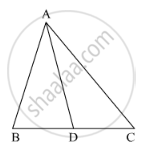# In the Following Figure, Check Whether Ad is the Bisector of ∠A of ∆Abc in the Following Case: Ab = 8 Cm, Ac = 24 Cm, Bd = 6 Cm and Bc = 24 Cm - Mathematics

In the following figure, check whether AD is the bisector of ∠A of ∆ABC in the following case:

AB = 8 cm, AC = 24 cm, BD = 6 cm and BC = 24 cm#### Solution

It is given that, AB = 8 cm, AC = 24 cm, BD = 6 cm and BC = 24 cm

We have to check whether AD is bisector of ∠A.

First we will check proportional ratio between sides.

Now

DC = BC - BD

DC = 24 - 6 = 18

So "AB"/"AC"="BD"/"DC"

rArr8/24=6/18

rArr1/3=1/3

(It is proportional)

Hence, AD is bisector of ∠A.

Concept: Angle Bisector
Is there an error in this question or solution?

#### APPEARS IN

RD Sharma Class 10 Maths
Chapter 7 Triangles
Exercise 7.3 | Q 4.3 | Page 31